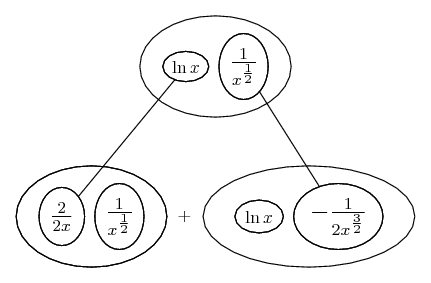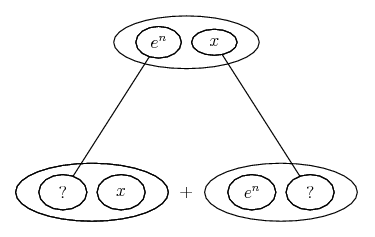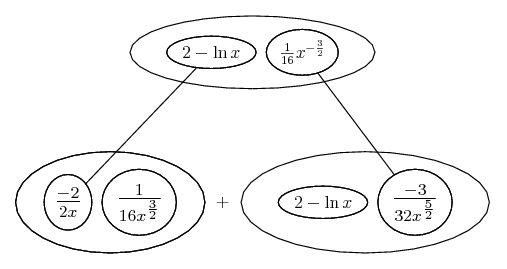# Thread: Where a function is increasing/decreasing?

1. ## Where a function is increasing/decreasing?

$\displaystyle F(x)=\frac{ln(x)}{8\sqrt{x}}$

We're supposed to find:

~The intervals on which f is increasing and decreasing
~The local maximum of f
~The inflection point
~The interval on which f is concave up
~The interval on which f is concave down

For the first one, I know that we're supposed to use the critical points to "divide" the function into invervals, but I don't understand it in terms of this function.

Even if you graph it, anything x <= 0 doesn't work, and if you take the derivative to find the critical points:

$\displaystyle F'(x) = \frac{f'(x)g(x) - f(x)g'(x)}{g(x)^2}$
f(x) = ln x
f'(x) = $\displaystyle \frac{1}{x}$
g(x) = $\displaystyle 8\sqrt{x}$
g'(x) = 4x^(-1/2) = $\displaystyle \frac{4}{\sqrt{x}}$

$\displaystyle F'(x) = \frac{\frac{8\sqrt{x}}{x} - \frac{4lnx}{\sqrt{x}}}{64x}$

Which I think raises a similar problem, because F'(x) is 0 at x = 0 and undefined x <= 0 because of the ln. So what am I supposed to do? I'm hoping that the rest of the problem is doable once the first bullet is taken care of, but I don't know.

2. $\displaystyle F(x) = \frac{\ln{x}}{8\sqrt{x}}$

domain of $\displaystyle F(x)$ is $\displaystyle x > 0$

$\displaystyle F'(x) = \frac{\frac{8\sqrt{x}}{x} - \frac{4\ln{x}}{\sqrt{x}}}{64x}$

multiply every term by $\displaystyle \sqrt{x}$ ...

$\displaystyle F'(x) = \frac{8 - 4\ln{x}}{64\sqrt{x^3}}$

$\displaystyle 8 - 4\ln{x} = 0$

$\displaystyle \ln{x} = 2$

$\displaystyle x = e^2$

so, the only critical value in the domain of $\displaystyle F(x)$ is
$\displaystyle x = e^2$

proceed with your analysis.

3. So f is undefined on the interval ]-inf, 0]. Well done. Carry on...

Life often simpler without the quotient rule (and especially if we cheat a bit by factoring out the constant 1/8)...... where... is the product rule. Straight continuous lines differentiate downwards (integrate up) with respect to x.

__________________________________________

Don't integrate - balloontegrate!

Balloon Calculus: Gallery

Balloon Calculus Drawing with LaTeX and Asymptote!

4.Originally Posted by skeeter$\displaystyle F(x) = \frac{\ln{x}}{8\sqrt{x}}$

domain of $\displaystyle F(x)$ is $\displaystyle x > 0$

$\displaystyle F'(x) = \frac{\frac{8\sqrt{x}}{x} - \frac{4\ln{x}}{\sqrt{x}}}{64x}$

multiply every term by $\displaystyle \sqrt{x}$ ...

$\displaystyle F'(x) = \frac{8 - 4\ln{x}}{64\sqrt{x^3}}$

$\displaystyle 8 - 4\ln{x} = 0$

$\displaystyle \ln{x} = 2$

$\displaystyle x = e^2$

so, the only critical value in the domain of $\displaystyle F(x)$ is
$\displaystyle x = e^2$

proceed with your analysis.
So I'm guessing that means that it's increasing on (0,e^2) and decreasing on (e^2, inf)?

@ Tom - Yes. I'm really growing to loathe the quotient rule.

5. No need to guess. Differentiate again...Fill in the blanks? Then see whether the second derivative is +ive or -ive at x = e^2.

6. Edit 2: About finding concave up/concave down, I know that I need to find F''(x).

$\displaystyle \frac{8 - 4\ln{x}}{64\sqrt{x^3}}$ = $\displaystyle (8-4lnx)(64\sqrt{x^3})^-1$
f(x) = 8-4lnx
f'(x) = $\displaystyle \frac{-4}{x}$
g(x) = $\displaystyle (64\sqrt{x^3})^-1$
g'(x) = (too much work to type out unless I get it wrong) = [tex]\frac{1}{128x^\frac{3}{2}}

F''(x) = $\displaystyle \frac{-4(64\sqrt{x^3})^-1}{x}+ \frac{8-4lnx}{128x^\frac{3}{2}}$

Now, I put this into my calculator, and It crosses the x axis somewhere between 7 and 8. How do I find that point? (assuming that this ridiculous derivative is correct)

@ Tom- Maybe I'm just delirious because I have H1N1, but where did those equations at the top come from?

7. If you'd simplified your F' I could maybe have followed your product rule working. Here is mine. Simplify yours to see if it matches...Bottom row simplifies to $\displaystyle \frac{3 \ln|x| - 8}{32 x^{\frac{5}{2}}}$

Now, you only want to set the second derivative to zero to find inflection points. At the moment you just want to see whether the curve is curving up (at a minimum) or down (at a maximum) when x is e^2. Curving up, the 2nd deriv is +ive, down... you get the idea.

8.Originally Posted by tom@ballooncalculusIf you'd simplified your F' I could maybe have followed your product rule working. Here is mine. Simplify yours to see if it matches...Bottom row simplifies to $\displaystyle \frac{3 \ln|x| - 8}{32 x^{\frac{5}{2}}}$

Now, you only want to set the second derivative to zero to find inflection points. At the moment you just want to see whether the curve is curving up (at a minimum) or down (at a maximum) when x is e^2. Curving up, the 2nd deriv is +ive, down... you get the idea.
I figured I could just take a short cut and set the second derivative to 0 and find the inflection point, and then after I have that finding concave up/down would be easier.

Is there a "proper" way to solve for x when y = 0? It looks like it's going to be atrocious if I just try to plug-in.

Sorry for all of this; I'm pretty slow this evening.9. Other way round - Plug in easy, solve hard! Just see whether your calculator says =(3*LOG(EXP(1)^2) - 8)/(32*(EXP(1)^5)) (or even simpler) is +ive or -ive

10. I did, which is sort of what prompted the question.

The inflection point/x intercept for F''(x) is between 14 and 15, but because of the way my assignments are graded, we usually don't get credit for decimal answers.

If there's no simpler solution to get, then I think I'm stuck.11. Sorry, you did want the inflection point as well... yes, just solve

$\displaystyle \frac{3 \ln x - 8}{32 x^{\frac{5}{2}}} = 0$

Are you thinking this is worse than it is? Multiply both sides by $\displaystyle 32 x^{\frac{5}{2}}$ ...

$\displaystyle 3 \ln x - 8 = 0$

etc

#### Search Tags

function, increasing or decreasing